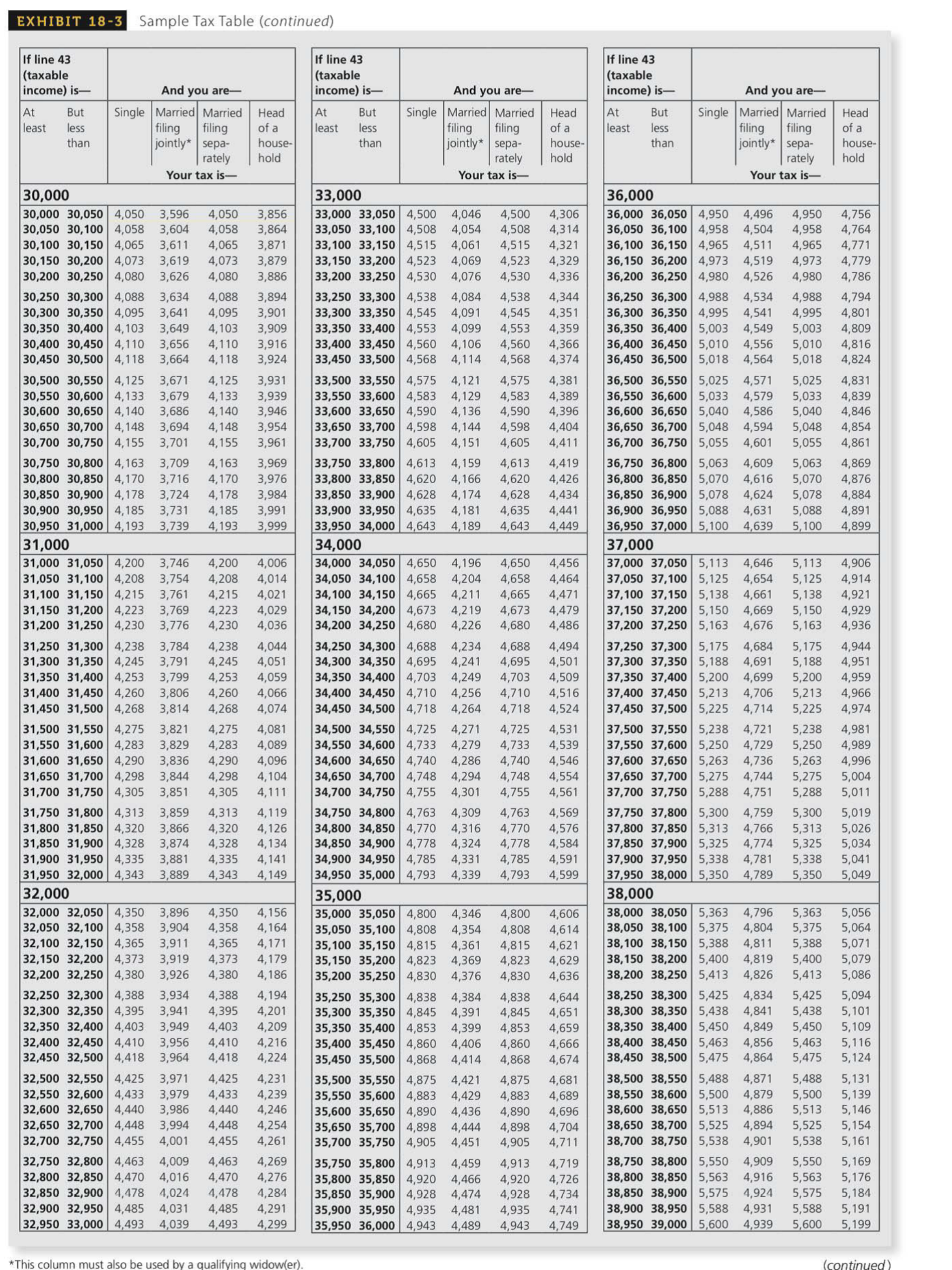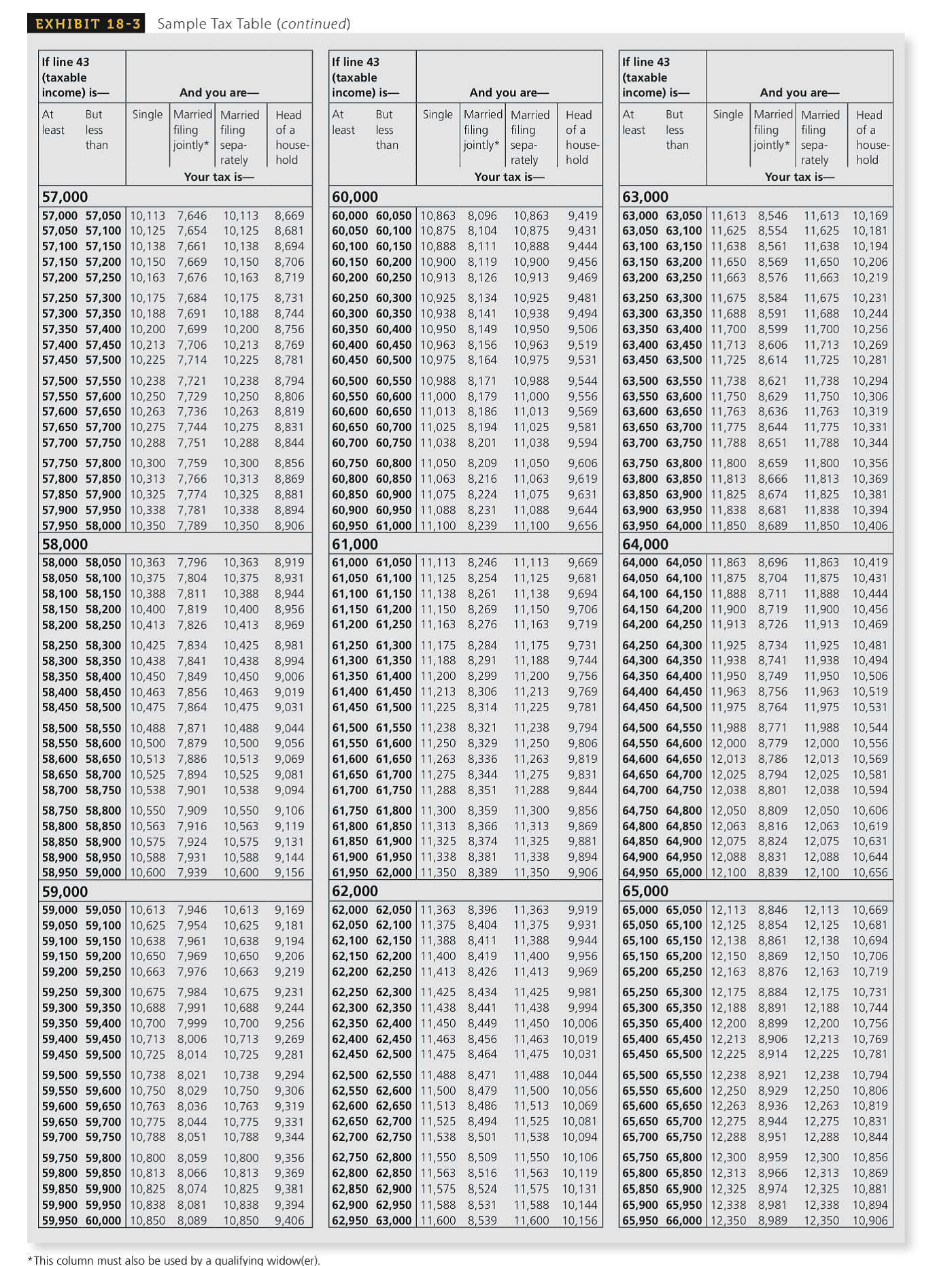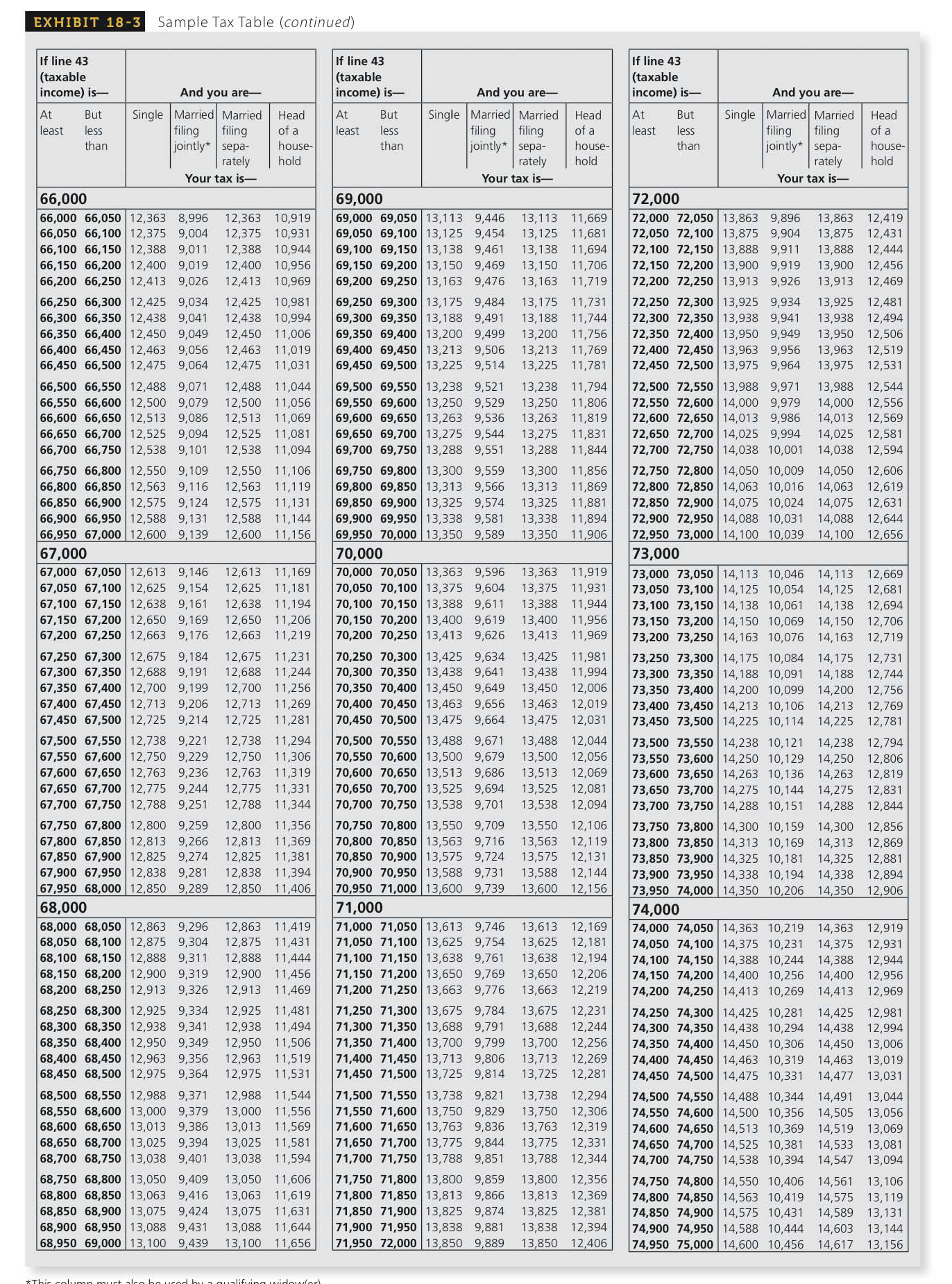Chapter 18.III, Problem 14RE### Contemporary Mathematics for Busin...

8th Edition
Robert Brechner + 1 other
ISBN: 9781305585447

#### Solutions

Chapter
Section### Contemporary Mathematics for Busin...

8th Edition
Robert Brechner + 1 other
ISBN: 9781305585447
Textbook Problem

# Use the Tax Table, Exhibit 18-3, to calculate the tax liability for the following taxpayers earning under $100,000.Name Filing Status Taxable Income Tax Liability 14. Rice Married, Jointly$69,915

To determine

To calculate: The tax liability for the tax payer Rice whose taxable income is $69,915. Explanation Given Information: The Person Rice is married and jointly filed with the taxable income of$69,915.

Calculation:

Taxable income is defined as the income to which tax rates are functional in order to discover the total amount of tax payable for the year. Tax table is defined as the table which is used to find the income tax whose earnings are less than \$100,000.

The followings steps are used to calculate,

Step 1: Open the tax table EXHIBIT 18-3 and it is needed to find the line which contains the amount of taxable income...

### Still sussing out bartleby?

Check out a sample textbook solution.

See a sample solution

#### The Solution to Your Study Problems

Bartleby provides explanations to thousands of textbook problems written by our experts, many with advanced degrees!

Get Started

#### 12. If , find the following. (a) (b) (c) (d)

Mathematical Applications for the Management, Life, and Social Sciences

#### Evaluate the indefinite integral. cos(lnt)tdt

Single Variable Calculus: Early Transcendentals, Volume I

#### limx(lnx)1/x= a) 0 b) 1 c) e d)

Study Guide for Stewart's Single Variable Calculus: Early Transcendentals, 8th